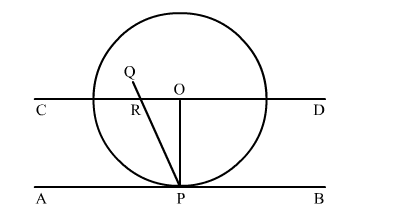# Prove that the perpendicular at the point of contact of the tangent to a circle passes through the centre.`
Question:

Prove that the perpendicular at the point of contact of the tangent to a circle passes through the centre.

Solution:Let AB be the tangent to the circle at point P with centre O.
To prove:  PQ passes through the point O.
Construction: Join OP.
Through O, draw a straight line CD parallel to the tangent AB.
Proof: Suppose that PQ doesn't passes through point O.
PQ intersect CD at R and also intersect AB at P.
AS, CD ∥ AB, PQ is the line of intersection,
∠ORP = ∠RPA (Alternate interior angles)
but also,
∠RPA = 90 (OP ⊥ AB)
⇒ ∠ORP  = 90
∠ROP + ∠OPA = 180 (Co interior angles)
⇒∠ROP + 90 = 180
⇒∠ROP = 90
Thus, the ΔORP has 2 right angles i.e. ∠ORP  and ∠ROP which is not possible.
Hence, our supposition is wrong.
∴ PQ passes through the point O.